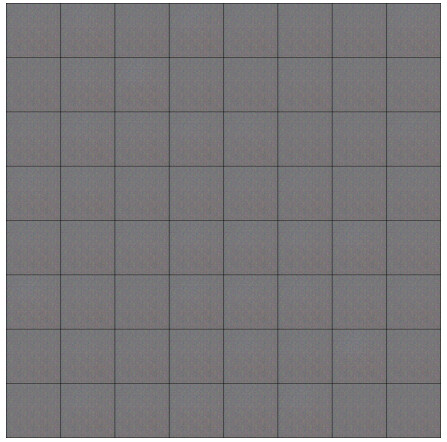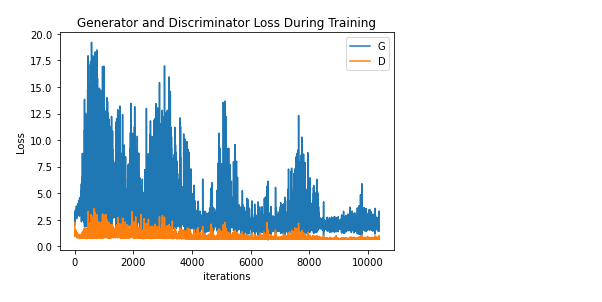# GAN Image Quality Very Low

I am trying to follow the DCGAN tutorial in order to train a GAN to generate Monet paintings from the following dataset. This dataset provides 3 x 256 x 256 images of Monet paintings.

The problem I am running in to is that the GAN results look very much like garbage images and as I am new to training GANs I am unsure if I need to simply train longer, sample from a larger latent space, increase the size of my models, or perform some similar tweak. I would love some input on what I can try to improve my model. I’ve included some code below.

This is what the set of images the Generator produces look like:Here is an image of the loss through training:I am using the following hyperparameters with ADAM optimizer for both Generator and Discriminator:

``````z_dim = 100 #noise dimensions
lr = 0.00001
betas = (.5, .999)
batch_size = 32
num_epochs = 200
``````

I’ve tried a few changes so far but would love some input on this.
Notes on training attempts:

1. Vanilla attempt, D loss goes to 100 G loss goes to 0 around epoch 17, seems like mode collapse. Generator produces noise images which somehow minimize loss

2. As we have only about 1800 samples the collapse in the previous iteration could be due to a high learning rate, in this experiment lets reduce the learning rate from 0.0002 to 0.00001 the intuition for this change is that the generator may be overfitting for this particular discriminator too quickly (this is supported by the fact that this occurs in an early epoch)

Here the training looked more stable but the discriminator loss went to 0 while the GAN loss stbailized around 45-50. This is a documented issue. It seems that the Discriminator is learning the real distribution faster than the Generator can fool it, the learning rate reduction certainly helped but the generator still produced garbage images.

1. To address the Discriminator’s strength relative to the Generator in the last experiment lets impair the Discriminator by implementing a form of label smoothing - adding noise to the labels so that the discriminators confidence decreases. Lets start with a simple technique: change real_label from 1->0.9 and fake_label from 0->0.1

This improves the GAN training and doesn’t cause the discriminator loss to go to 0 as before, lets increase num_epochs from 50->200

Even with these changes the model still produces images of low quality like what I showed above.

These are the models:

``````Generator(
(network): ModuleList(
(0): Sequential(
(0): ConvTranspose2d(100, 2048, kernel_size=(4, 4), stride=(1, 1), bias=False)
(1): BatchNorm2d(2048, eps=1e-05, momentum=0.1, affine=True, track_running_stats=True)
(2): ReLU(inplace=True)
)
(1): Sequential(
(0): ConvTranspose2d(2048, 1024, kernel_size=(4, 4), stride=(2, 2), padding=(1, 1), bias=False)
(1): BatchNorm2d(1024, eps=1e-05, momentum=0.1, affine=True, track_running_stats=True)
(2): ReLU(inplace=True)
)
(2): Sequential(
(0): ConvTranspose2d(1024, 512, kernel_size=(4, 4), stride=(2, 2), padding=(1, 1), bias=False)
(1): BatchNorm2d(512, eps=1e-05, momentum=0.1, affine=True, track_running_stats=True)
(2): ReLU(inplace=True)
)
(3): Sequential(
(0): ConvTranspose2d(512, 256, kernel_size=(4, 4), stride=(2, 2), padding=(1, 1), bias=False)
(1): BatchNorm2d(256, eps=1e-05, momentum=0.1, affine=True, track_running_stats=True)
(2): ReLU(inplace=True)
)
(4): Sequential(
(0): ConvTranspose2d(256, 128, kernel_size=(4, 4), stride=(2, 2), padding=(1, 1), bias=False)
(1): BatchNorm2d(128, eps=1e-05, momentum=0.1, affine=True, track_running_stats=True)
(2): ReLU(inplace=True)
)
(5): Sequential(
(0): ConvTranspose2d(128, 64, kernel_size=(4, 4), stride=(2, 2), padding=(1, 1), bias=False)
(1): BatchNorm2d(64, eps=1e-05, momentum=0.1, affine=True, track_running_stats=True)
(2): ReLU(inplace=True)
)
(6): Sequential(
(0): ConvTranspose2d(64, 3, kernel_size=(4, 4), stride=(2, 2), padding=(1, 1), bias=False)
(1): Tanh()
)
)
)

Discriminator(
(network): ModuleList(
(0): Sequential(
(0): Conv2d(3, 64, kernel_size=(4, 4), stride=(2, 2), padding=(1, 1), bias=False)
(1): LeakyReLU(negative_slope=0.2, inplace=True)
)
(1): Sequential(
(0): Conv2d(64, 128, kernel_size=(4, 4), stride=(2, 2), padding=(1, 1), bias=False)
(1): BatchNorm2d(128, eps=1e-05, momentum=0.1, affine=True, track_running_stats=True)
(2): LeakyReLU(negative_slope=0.2, inplace=True)
)
(2): Sequential(
(0): Conv2d(128, 256, kernel_size=(4, 4), stride=(2, 2), padding=(1, 1), bias=False)
(1): BatchNorm2d(256, eps=1e-05, momentum=0.1, affine=True, track_running_stats=True)
(2): LeakyReLU(negative_slope=0.2, inplace=True)
)
(3): Sequential(
(0): Conv2d(256, 512, kernel_size=(4, 4), stride=(2, 2), padding=(1, 1), bias=False)
(1): BatchNorm2d(512, eps=1e-05, momentum=0.1, affine=True, track_running_stats=True)
(2): LeakyReLU(negative_slope=0.2, inplace=True)
)
(4): Sequential(
(0): Conv2d(512, 1024, kernel_size=(4, 4), stride=(2, 2), padding=(1, 1), bias=False)
(1): BatchNorm2d(1024, eps=1e-05, momentum=0.1, affine=True, track_running_stats=True)
(2): LeakyReLU(negative_slope=0.2, inplace=True)
)
(5): Sequential(
(0): Conv2d(1024, 2048, kernel_size=(4, 4), stride=(2, 2), padding=(1, 1), bias=False)
(1): BatchNorm2d(2048, eps=1e-05, momentum=0.1, affine=True, track_running_stats=True)
(2): LeakyReLU(negative_slope=0.2, inplace=True)
)
(6): Sequential(
(0): Conv2d(2048, 1, kernel_size=(4, 4), stride=(1, 1), bias=False)
(1): Sigmoid()
)
)
)
``````

Here is the training loop:

``````# Training Loop - adapted directly from DCGAN tutorial

# Lists to keep track of progress
img_list = []
G_losses = []
D_losses = []
iters = 0

num_epochs = 200

real_label = 0.9
fake_label = 0.1

print("Starting Training Loop...")
print(device)
# For each epoch
for epoch in range(num_epochs):
# For each batch in the dataloader
for i, data in enumerate(dataloader, 0):

############################
# (1) Update D network: maximize log(D(x)) + log(1 - D(G(z)))
###########################
## Train with all-real batch
# Format batch
real_cpu = data.to(device)
b_size = real_cpu.size(0)
label = torch.full((b_size,), real_label, dtype=torch.float, device=device)
# Forward pass real batch through D
output = netD(real_cpu).view(-1)
# Calculate loss on all-real batch
errD_real = criterion(output, label)
# Calculate gradients for D in backward pass
errD_real.backward()
D_x = output.mean().item()

## Train with all-fake batch
# Generate batch of latent vectors
noise = torch.randn(b_size, z_dim, 1, 1, device=device)
# Generate fake image batch with G
fake = netG(noise)
label.fill_(fake_label)
# Classify all fake batch with D
output = netD(fake.detach()).view(-1)
# Calculate D's loss on the all-fake batch
errD_fake = criterion(output, label)
# Calculate the gradients for this batch
errD_fake.backward()
D_G_z1 = output.mean().item()
errD = errD_real + errD_fake
# Update D
optimizerD.step()

############################
# (2) Update G network: maximize log(D(G(z)))
###########################
label.fill_(real_label)  # fake labels are real for generator cost
# Since we just updated D, perform another forward pass of all-fake batch through D
output = netD(fake).view(-1)
# Calculate G's loss based on this output
errG = criterion(output, label)
errG.backward()
D_G_z2 = output.mean().item()
# Update G
optimizerG.step()

# Output training stats
if i % 50 == 0:
print('[%d/%d][%d/%d]\tLoss_D: %.4f\tLoss_G: %.4f\tD(x): %.4f\tD(G(z)): %.4f / %.4f'
errD.item(), errG.item(), D_x, D_G_z1, D_G_z2))

# Save Losses for plotting later
G_losses.append(errG.item())
D_losses.append(errD.item())

# Check how the generator is doing by saving G's output on fixed_noise
if (iters % 500 == 0) or ((epoch == num_epochs-1) and (i == len(dataloader)-1)):
fake = netG(fixed_noise).detach().cpu()

iters += 1
``````

I would seriously appreciate any help, it would be great to know if I’m on the right track and what some more experienced people have to think about the problem. It is worth noting that the dataset itself has about 1800 images in it, and some of those paintings are quite distinct - intuitively this could cause a problem for the model, is this something I should focus on remedying or can I get around it? Thank you!

When the program runs this line of code, the gradient of the network for discriminating real images is totally discarding.

Here is some code for reference:

``````self.pred_fake = self.netD(fake_B.detach())
self.pred_real = self.netD(real_B)
self.loss_D_fake = self.criterionGAN(self.pred_fake, False)
self.loss_D_real = self.criterionGAN (self.pred_real, True)

self.loss_D = (self.loss_D_fake + self.loss_D_real) * 0.5
self.loss_D.backward()
``````

Thanks for the reply! I did think that this snippet was strange, but I pulled it directly from the DCGAN tutorial so I figured it would work. I made the change below:

``````# Training Loop - adapted directly from DCGAN tutorial

# Lists to keep track of progress
img_list = []
G_losses = []
D_losses = []
iters = 0

num_epochs = 200

real_label = 0.9
fake_label = 0.1

print("Starting Training Loop...")
print(device)
# For each epoch
for epoch in range(num_epochs):
# For each batch in the dataloader
for i, data in enumerate(dataloader, 0):

############################
# (1) Update D network: maximize log(D(x)) + log(1 - D(G(z)))
###########################
## Train with all-real batch
# Format batch
real_cpu = data.to(device)
b_size = real_cpu.size(0)
label = torch.full((b_size,), real_label, dtype=torch.float, device=device)
# Forward pass real batch through D
output_real = netD(real_cpu).view(-1)

# Calculate gradients for D in backward pass
#errD_real.backward()
D_x = output.mean().item()

## Train with all-fake batch
# Generate batch of latent vectors
noise = torch.randn(b_size, z_dim, 1, 1, device=device)
# Generate fake image batch with G
fake = netG(noise)
f_label = torch.full((b_size,), fake_label, dtype=torch.float, device=device)#.fill_(fake_label)
# Classify all fake batch with D
output_fake = netD(fake.detach()).view(-1)

# Calculate the gradients for this batch
#errD_fake.backward()

# Calculate loss on all-real batch
errD_real = criterion(output_real, label)
# Calculate D's loss on the all-fake batch
errD_fake = criterion(output_fake, f_label)

errD = errD_real + errD_fake
#calculate average gradient as per Naruto-Sasuke

D_G_z1 = output.mean().item()
# Update D
optimizerD.step()

############################
# (2) Update G network: maximize log(D(G(z)))
###########################
label.fill_(real_label)  # fake labels are real for generator cost
# Since we just updated D, perform another forward pass of all-fake batch through D
output = netD(fake).view(-1)
# Calculate G's loss based on this output
errG = criterion(output, label)
errG.backward()
D_G_z2 = output.mean().item()
# Update G
optimizerG.step()

# Output training stats
if i % 50 == 0:
print('[%d/%d][%d/%d]\tLoss_D: %.4f\tLoss_G: %.4f\tD(x): %.4f\tD(G(z)): %.4f / %.4f'
errD.item(), errG.item(), D_x, D_G_z1, D_G_z2))

# Save Losses for plotting later
G_losses.append(errG.item())
D_losses.append(errD.item())

# Check how the generator is doing by saving G's output on fixed_noise
if (iters % 500 == 0) or ((epoch == num_epochs-1) and (i == len(dataloader)-1)):